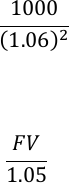Class Notes (1,100,000)
CA (650,000)
WLU (20,000)
BU (4,000)
BU473 (10)
Lecture 15

# BU473 Lecture Notes - Lecture 15: Yield Curve, Arbitrage, Investment

Department
Course Code
BU473
Professor
David Cimon
Lecture
15

This preview shows page 1. to view the full 4 pages of the document.BU-473 Lecture 15
Yield Curve
Describes how a bond’s term to maturity varies based on its yield
Shape of the yield curve describes the current state of fixed income market
o Upwards slope means bonds with longer maturities pay higher yields
o Downwards slope means bonds with longer maturities pay lower yields
On-the-run vs. Pure Yields
Most bonds have cash flows that take place over different periods of time
To calculate the pure yield for a given period of time, we only want the bond to have cash flows
at that exact period of time
The pure yield curve is imputed by taking government treasuries, zero-coupon bonds and strips
with different maturities and imputing a curve
Alternatively, an on-the-run yield curve is imputed by taking on-the-run coupon bonds near their
pay values
These 2 curves can have substantially different values
Yield Curve and Future Rates
Yield curve can provide info about the market expectations of future interest rate changes
In equilibrium, we cannot have arbitrage, we need to have equal cash flows between 2
strategies:
o Buying and holding a bond for a certain period of time
o Buying, holding until maturity, and reinvesting in a series of shorter-term bonds over
that same period of time
Consider two bonds:
o A 2 year zero-coupon bond with a yield of 6%.
o A 1 year zero-coupon bond with a yield of 5%.
If we want to buy \$1,000 in face value of the 2 year bond, it will cost:
𝑃2=1000
(1.06)2= \$890.00
If we invested that \$890 into the 1 year bond instead, we would receive the following face value
after 1 year:
890.00 =𝐹𝑉
1.05 𝐹𝑉 = \$934.50
For there to be no arbitrage in the 2nd year, we must be able to reinvest that \$934.50 from the
1st year and earn exactly \$1,000 total. For that to happen, the 1-year yield in year 2 would have
to be: